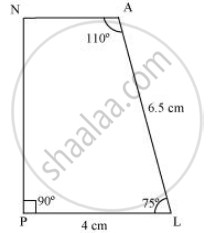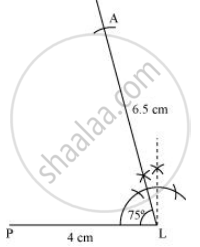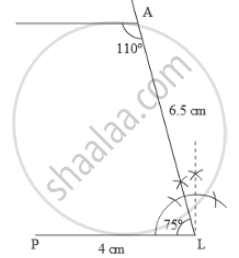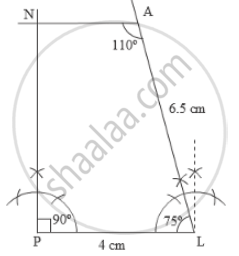Share

# Construct the Following Quadrilaterals. Quadrilateral Plan Pl = 4 Cm La = 6.5 Cm ∠P = 90° ∠A = 110° ∠N = 85° - CBSE Class 8 - Mathematics

ConceptConstructing a Quadrilateral When Two Adjacent Sides and Three Angles Are Known

PL = 4 cm

LA = 6.5 cm

∠P = 90°

∠A = 110°

∠N = 85°

#### Solution

1) The sum of the angles of a quadrilateral is 360°.

In quadrilateral PLAN, ∠P + ∠L + ∠A + ∠N = 360°

90° + ∠L + 110° + 85° = 360°

285° + ∠L = 360°

∠L = 360° − 285° = 75°

2) A rough sketch of this quadrilateral is as follows.3) Draw a line segment PL of 4 cm and draw an angle of 75º at point L. As vertex A is 6.5 cm away from vertex L, cut a line segment LA of 6.5 cm from this ray4) Again draw an angle of 110º at point A.5) Draw an angle of 90º at point P. This ray will meet the previously drawn ray from A at point N.